# Using Geometry Values with GridDB

Data comes in many, many forms. It comes temporally as well as spatially. GridDB’s Timeseries containers as well as its TQL and time-specific API operations cover the time aspect of data. GridDB has geospatial data covered as well in GridDB Standard Edition and Advanced Edition. Support is provided with Geometry Columns and Data Types along with specialized Geometry Operations through TQL as well as through the C and Java APIs.

In GridDB, Geometry values can be created to represent 5 general models or shapes.

# POINT

A coordinate point in either two dimensional or three dimensional space.
2D and 3D Points

`Geometry point = Geometry.valueOf("POINT(1 3.4)"); Geometry threeDPt = Geometry.valueOf("POINT(2 -3 0.43)");`

# LINESTRING

A set of one or more interconnected lines in two or three dimensional space. They are represented by two or more points.

# POLYGON

A closed area in either two or three dimensional space. The area consists of three or more points that are connected from a starting point, or `VERTEX`, to the endpoints and back to the starting point. It is possible to have the surface or area to have gaps or islands that are not part of the model by specifying the internal areas to exclude from the outer area.
2D Polygon

`Geometry polygon = Geometry.valueOf("POLYGON((0 0, 1 0, 1 1, 0 1, 0 0))");`

3D Polygon

`Geometry threeDSurface = Geometry.valueOf("POLYGON((0 0 0, 1 0 1, 1 1 1, 0 1 0, 0 0 0))");`

# POLYHEDRALSURFACE

The `POLYHEDRALSURFACE` surface consists of vertices, edges, and facets. Simple examples tetrahedrons, polyhedrons, and octohedral surfaces. The POLYHEDRALSURFACE can only be a three-dimensional shape. This means all the points that make up its edges are supposed to contain 3 coordinates. Polyhedral surfaces can be thought of as a full three-dimensional extension of the POLYGON in that the shape can enclose a volume where its faces are each comprised of a closed polygonal surface.
Example

`Geometry polySurface = Geometry.valueOf( \ "POLYHEDRALSURFACE ( ((0 0 0, 0 1 0, 1 1 0, 1 0 0, 0 0 0)),"+ "((0 0 0, 0 1 0, 0 1 1, 0 0 1, 0 0 0 ))," + "((0 0 0, 1 0 0, 1 0 1, 0 0 1, 0 0 0))," + "((1 1 1, 1 0 1, 0 0 1, 0 1 1, 1 1 1)) ," + "((1 1 1, 1 0 1, 1 0 0, 1 1 0, 1 1 1))," + "((1 1 1, 1 1 0, 0 1 0, 0 1 1, 1 1 1)) )");`

Every set of coordinates should be enclosed by double-parenthesis `((` , `))` because it represents a closed face that will make up part of the three-dimensional enclosed surface. Think of the faces as `POLYGON`‘s that make up the sides of the surface. All these are then grouped and connected together to form the three-dimensional surface object.

A three dimensional quadratic surface. These objects are curved surfaces in three-dimensional space by the equation: `f(X) = <AX,X> + BX + C`. Create a surface by inserting 16 numbers that represent the coefficents of making that surface equation.
The QUADRATICSURFACE is different from the other four Geometry objects in that its WKT (Well-Known-Text format) inputs are coefficients to an equation rather than coordinates in the 3D plane. This equation,

`ax2+by2+cz2+2fyz+2gzx+2hxy+2px+2qy+2rz+d = 0`

Example Surface

`GSRow* row; const GSChar* wkt = "QUADRATICSURFACE(( 84 2 3 5 6 7 8 9 10 12 13 14 15 16 17 18 ))"; // (snip)gsGetNextRow(rowSet,row); gsSetRowFieldByGeometry(row,4,wkt); gsPutRow(timeseries,NULL,row,NULL);`

Examples of quadric surfaces include parallel planes, hyperboloids, cones, cylinders and spheres. These types of surfaces are mapped to different polynomial equations. Some of these equations may not have certain factors or coefficients. For example, there may be no `x`‘s in the equation like in

`y2+ z2- 1`

Since there are no x’s in the equation, replace all coefficients that are near x like a,h,p with 0’s. When entering the matrix values for the QUADRATICSURFACE WKT, there must always be 16 values, with any missing values replaced with a 0.

More examples and information on quadric surfaces can be found in this Wolfram Alpha page.

# Creating Objects with WKT Format

Creating any of these objects in GridDB is fairly simple. All that is needed on the development end is to know how to establish the model in `WKT` form. WKT (Well-Known-Text) text formatting is a text markup language used for representing vector geometry objects on a map or in a spatial reference system. If the WKT string is parsed correctly, the Geometry is created and inserted into its respective column into the Collection or Timeseries. There are certain characteristics of WKT format that should be noted and followed or else errors will be thrown or the Geometry object will not be created.

General Formatting Rules

When stating the shape of the Geometry (POINT,LINESTRING,etc.) ensure that you use ALL UPPERCASE LETTERS and correct syntax. If not done so, the object will not be created and exceptions could be raised. Ensure that the formatting is correct and there are no syntax errors such as not having correct amount of parenthesis or not using proper spacing.

If you wish to create an empty Geometry object, create a `POINT(EMPTY)` geometry.
Empty LINESTRING and POLYGON

`Geometry emptyPoint = Geometry.valueOf("POINT(EMPTY)");`

Range Restrictions

In some GIS-supporting (Geographic Information System) databases, the ranges or values of points can include special values like `NaN`, `INF`, `-INF` (Not-a-Number, Negative and Positive Infinity). Only numerical-types can be used which include positive or negative floating point numbers.

Forming Closed Surfaces

Usually Geometries beyond POINT and QUADRATICSURFACE consist of points that are connected. The points, once connected together, form lines that will make up the edges. The edges usually converge to form a closed surface. For all Geometries except POINT, more than one data point is needed.

Dimensions of Geometry Values

The points that make a Geometry can only be in two-dimensional or three-dimensional format. Also, when creating points to outline an object the dimensions must stay consistent (i.e a polygon that has a coordinate in 2D must have all its other coordinates in 2D). Points are separated by commas: `,` and the `x,y,z` coordinates are delimited by `SPACES`

# Defining Geometric Columns and Indexes

Geometry columns can be added to the column schema for a container either in a static fashion or a dynamic fashion. The `Geometry` can be directly imported from the GridDB library and can be set as a column type for a class schema for a container. You can also set an index on a Geometry column by setting a `SPATIAL` index flag.
Java Container Creation

`// (snip)import com.toshiba.mwcloud.gs.Geometry;import com.toshiba.mwcloud.gs.GeometryOperator;//(snip)static class Shape {	@RowKey String name;	double perimeter;	double volume;	Date creation;	Geometry geom;	public String toString(){		return String.format("Shape: Name = %s, Perimeter = %f, \                                   Volume = %f, Creation Date = %s, WKT = %s",\                                 name,perimeter,volume,creation.toString(),geom.toString());//(snip)Collection<String,Shape> geometryCollection = \               gridstore.putCollection("GeometryCollection101",Shape.class);// (snip)geometryCollection.createIndex("geom",IndexType.SPATIAL);`

There is no explicit GSGeometry struct or enum in GridDB’s C API. When creating a container with a dynamic schema using `ContainerInfo` and `ColumnInfo` objects, set the ColumnInfo’s type as `GS_TYPE_GEOMETRY`. If you are using struct-bindings, set the column type that you wish to set as Geometry to have the type `const GSChar*`. From there, set the column’s type to Geometry when performing the struct-bindings. Simply set the column value with its WKT form and GridDB will convert it to its binary equivalent in the database.
C Container Creation

`typedef struct {	const GSChar* name;	GSTimestamp timestamp;	double volume;	double perimeter;	const GSChar* shape;} GeometryRow;GS_STRUCT_BINDING(GeometryRow,	GS_STRUCT_BINDING_KEY(name, GS_TYPE_STRING)	GS_STRUCT_BINDING_ELEMENT(timestamp,GS_TYPE_TIMESTAMP)	GS_STRUCT_BINDING_ELEMENT(volume,GS_TYPE_DOUBLE)	GS_STRUCT_BINDING_ELEMENT(perimeter,GS_TYPE_DOUBLE)	GS_STRUCT_BINDING_ELEMENT(shape,GS_TYPE_GEOMETRY));//(snip)GSCollection* collection;gsPutCollection(gridstore,"GeometryCollection311", \GS_GET_STRUCT_BINDING(GeometryRow),NULL,GS_FALSE,&collection);gsCreateIndex(collection,"shape",GS_INDEX_FLAG_SPATIAL);GeometryRow gRow; gRow.name = "FirstPoint";gRow.timestamp = gsCurrentTime();gRow.volume = 0;double x, y, z;sscanf(wkt,"POINT(%lf %lf %lf)",&x,&y,&z);gRow.perimeter = sqrt((x * x) + (y * y) + (z * z));gRow.shape = "POINT(1 4.23 4.2)";gsPutRow(collection,NULL,&gRow,NULL);`

# Spatial Operations in GridDB’s API

Geometric data values, when inserted into a GridDB database, are stored in binary form, much like `BLOB` data types. This means that performing direct equality operations are not allowed with `TQL`.
Example of Invalid TQL

`SELECT * WHERE geom = ST_GeomFromText('POINT(2 4 5)') #(Throws Error)`

In Java it is possible to compare the Geometry values directly to each using the API from GridDB.

`Geometry pointToFind = Geometry.valueOf("POINT(0 4)");// (snip)while(rowSet.hasNext){     Row row = rowSet.next();     Geometry rowPoint = row.getGeometry(4);     boolean match = rowPoint.equals(pointToFind);     System.out.println(match);`

One spatial operation that is allowed through GridDB’s API and TQL is a `SPATIAL INTERSECTION`. This translates to searching for a common area or volume between the two objects. A 3D intersection consists of determining a Minimum Bounding Box, known as a rectangular parallelepiped, where the two objects intersect. When objects are two-dimensional, the z-coordinates have range values of `(-INF,INF)`. This means that checking against a 2D dimensional object with a 3D dimensional object will have a more inclusive z-range to increase the chances of intersection.

When comparing an object with a POINT, the bounding box’s vertices are made up of the points. The box’s vertices are all on the same side and have a length of 0. If comparing a POINT to a non-point object, GridDB will check if the bounding box contains the point in its area.

When comparing between shapes that are not points, such as two POLYGON objects, the bounding box will have minimum and maximum x, y, and z coordinates that will make up the intersection’s spatial structure.

Examples of Spatial Intersections

# Issuing Geometric Queries with GridDB API

Intersection queries can be made quickly without TQL on `Collection` containers by issuing `.query(String column,Geometry geom,GeometryOperator operator)` in Java or `gsQueryByGeometry()`. This query will search and fetch all rows
whose ‘column’ value will intersect with `const GSChar* geometry`.
Java

`Geometry intersection = Geometry.valueOf("POLYGON((0 0 0, 2 0 0, 2 2 0, 0 2 0, 0 0 0))");Query query = geometryCollection.query("geom",intersection, \ GeometryOperator.INTERSECT);RowSet rowSet = query.fetch(false);while(rowSet.hasNext()){	Shape shape = rowSet.next();	System.out.println(shape);`

C

`GSQuery* query;GSRowSet* rowSet;GeometryRow geometryRow;const GSChar* point = "POINT(1 4.56 4.2)";gsQueryByGeometry(collection,"shape",point,GS_GEOMETRY_OPERATOR_INTERSECT,&query);gsFetch(query,GS_FALSE,&rowSet);while(gsHasNextRow(rowSet)){	gsGetNextRow(rowSet, &fetchedRow);	GSChar timeStr[GS_TIME_STRING_SIZE_MAX];	gsFormatTime(fetchedRow.timestamp,timeStr,sizeof(timeStr));	printf("Name = %s",fetchedRow.name);	printf(" Timestamp = %s",timeStr);	printf(" Volume = %.2lf", fetchedRow.volume);	printf(" Perimeter = %.2lf", fetchedRow.perimeter);	printf(" Shape = %s\n",fetchedRow.shape);`

# TQL Queries with Geometry Values

TQL can be used to check for geometric intersections along with other conditions you wish to specify. In the case of comparing two non-quadratic shapes, you can use the GIS function `ST_MBRIntersects(geometry1,geometry2)`. If you wish to specify a Geometry to check for an intersection, simply enter the shape in WKT form and turn it into a Geometry object with TQL’s `ST_GeomFromText(wkt)` function.

Let’s say we want to fetch up to 5 rows whose ‘geom’ column value intersects with POLYGON:
`POLYGON((2 2 4, 5 2 4, 5 2 8, 2 2 8, 2 2 4))` AND whose value column is greater than 7.5 in descending order. You could issue the query as follows:

`String tql = "SELECT * WHERE ST_MBRIntersects(geom," +  "ST_GeomFromText('POLYGON((2 2 4, 5 2 4, 5 2 8, 2 2 8, 2 2 4))'))"+ " AND value > 7.5 ORDER BY value DESC LIMIT 5";Query<Row> query = collection.query(tql);`

One thing to note about ST_MBRIntersects is that you cannot use QUADRATICSURFACES for comparison. In the case that you want to check for intersection between a quadratic surface and a non-quadratic-surface. You can use the `ST_QSFMBRIntersects(quadratic,geometry)` to check for the intersection and use `ST_MakeQSF(A00,A10,A20,A01...)` The A00, A10, A20,A01 are used to represent the coefficents of the `<AX,X> + BX + C` equation that make up the Quadratic Surface.

`GSQuery* query;GSRowSet* rowSet;gsQuery(timeseries, \  "select * where STQSFMBRIntersects( \ST_MakeQSF(84,2,3,5,6,7,8,9,10,12,13,14,15,16,17,18),geom) AND id='row1'",\ &query);gsFetch(query,GS_FALSE,&rowSet);`

# Best Uses of Spatial Data

Geometric and spatial data types are highly useful in domains like engineering, science, architecture, geography, and IoT. In geography or IoT, spatial data can be used to represent latitude, longitude, and elevation of sensors or objects. On example could be the last three categories can be represented as three-dimensional point in the format `POINT(latitude longitude elevation)`. You can use spatial intersections with POINT’s to find a row at a specific location.

POLYGON‘s can be used to find rows that are within a geographic range. For example, a geometric query can be used with `POLYGON(57 110, 59 110, 59 130, 57 130, 57 110)` to find all rows within the area between 57 and 59 latitude and between 130 and 110 longitude.

Linestrings, polygons, and surfaces can be used to represent vectors or three dimensional object. Being able to create, store, query, and index spatial data is especially useful for fields like engineering and architecture where buildings and devices are based off of 3D designs.

A specific use case for GridDB could involve creating a collection of traffic sensors. It can store and track general data like whether its active or how many cars it has seen and other values. You can store its geographic location in terms of a POINT with the GEOMETRY. Intersection queries can be used to find filter based on locations using either API functions or TQL.

Traffic Sensor in Java

`public class TrafficLight {	@RowKey	public  String tag; // Sensor Id of Smart Traffic Light		public Geometry point; // Latitude, Longitude, and Elevation of Sensor	public Date installation; // Time of installation to the Traffic Grid	public boolean active; // Whether the traffic light sensor has been activated	public int reports; // Number of reports that the Traffic Light has issued	public String streets; // Cross streets of Traffic Light// (snip)`

Querying the Traffic Collection in Java

`Collection<String,TrafficLight> trafficLightCollection = \   gridstore.putCollection("trafficLights101",TrafficLight.class);// (snip)trafficLightCollection.createIndex("point",IndexType.SPATIAL);// (snip)// Search for a specific POINTString ptTql = "SELECT * WHERE ST_MBRIntersects(point," +                "ST_GeomFromText('POINT(47.33 84.72 0.7)'))";Query<TrafficLight> ptQuery = trafficLightCollection.query(ptTql);// (snip)// Search for Point in a 2D Range using POLYGONString tql = "SELECT * WHERE ST_MBRIntersects(point," +             "ST_GeomFromText('POLYGON((49.00 86.00, 51.00 86.00," +             "51.00 87.00, 49.00 87.00, 49.00 86.00))'))";Query<TrafficLight> query = trafficLightCollection.query(tql);`

# Using GridDB Standard Edition

In GridDB Community edition it is possible to create Geometry objects but it is not possible to insert them as column values or use them as part of a container’s schema. These features are only available for Standard Edition and beyond. GridDB Standard Edition can be used with AWS. General setup and configuration information can be found in the Quick Start Guide.

More information on the GEOMETRY type can be found in this section of the GridDB SE Technical Reference. There are more details on Spatial operations in the API Reference manual. GridDB uses regular expressions patterns to determine if an enter format is valid WKT. The patterns that are acceptable can be found in this section of the GridDB source code.

--

--

--

## More from GridDB

GridDB is a highly scalable, in-memory NoSQL time series database optimized for IoT and Big Data.

## SSTIC 2018 Part 2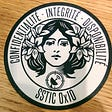## Python With Docker | FastAPI [Part-1]## Blog Post #8 — Final Project, CS108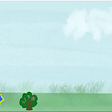## Learn Python in 10 minutes tutorial## Blue Goji capstone team: Finding alternative resources and solutions (2/3)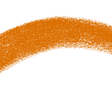## Finding Objects In Document Images## Do you want it right or do you want it right now?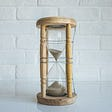## Image Classification using SingleStore DB, Keras and Tensorflow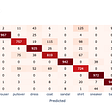## Surveying scikit-learn usage — Part III: Clustering and Overall Popularity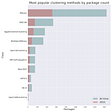## Dealing with Python Packages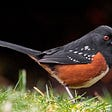## Query MS Graph API in Python